# NETWORKDAYS Excel FunctionArticle byExcelMojo Team## What Is NETWORKDAYS Function In Excel?

The NETWORKDAYS function in Excel calculates the total working days between any two given dates, excluding the weekends and specified holiday. This function is used to calculate employee settlements based on the tenure. The NETWORKDAYS Excel function is an inbuilt function so that we can insert the formula from the “Function Library” or enter it directly in the worksheet.

For example, the below table contains a list of start dates, end dates, and a holiday list in the Date format. We will count the working dates, excluding weekends and holidays.

Enter the formulas as follows:

• =NETWORKDAYS(A2,B2) in cell D2.
• =NETWORKDAYS(A3,B3) in cell D3.
• =NETWORKDAYS(A4,B4,C4) in cell D4.
• =NETWORKDAYS(A5,B5,C5:C7) in cell D5.

The output is shown above. D2 and D3 without entering the holidays argument. On the other hand, D4 and D5 exclude the specified holidays and weekends. Column E, for our reference, shows the formulas used in column D.

###### Key Takeaways
• The NETWORKDAYS Excel function calculates the total workdays between two given dates, excluding weekends and specified holidays.
• If we want to exclude the specified holidays from the workday count that fall on weekends, then the NETWORKDAYS() counts them as weekends, thus excluding only one instance of such dates from the workday count calculation.
• We can use the NETWORKDAYS() with other Excel functions such as EOMONTH, DATE(), and IF().
• If the start_date falls after the end_date, i.e., if the end_date precedes the start_date, then the NETWORKDAYS Excel function returns a negative number.

### NETWORKDAYS() Excel Formula

The syntax of the NETWORKDAYS Excel formula is:

The arguments of the NETWORKDAYS Excel formula are:

• start_date: A date representing the initial date. It is a mandatory argument.
• end_date: A date representing the end date. It is a mandatory argument.
• holidays: One or more holidays to exclude while calculating the work days. It is an optional mandatory argument.

### How to Use NETWORKDAYS Excel Function?

We can use the NETWORKDAYS Excel Function in 2 methods, namely,

1. Access from the Ribbon in Excel.
2. Enter in the worksheet manually.

#### Method #1 – Access from the Excel ribbon

First, choose an empty cell → select the “Formulas” tab → go to the “Function Library” → click the “Date & Time” option drop-down → select the “NETWORKDAYS” function, as shown below.

The “Function Arguments” window appears. Enter the argument values in the “Start_date”, “End_date”, and “Holidays” fields → click “OK”, as shown below.

#### Method #2 – Enter in the worksheet manually

First, ensure the date values are in Date format in the source data.

1. Select an empty cell.
2. Type =NETWORKDAYS( in the cell. [Alternatively, type =N and select the NETWORKDAYS function from the Excel suggestions.]
3. Enter the arguments as values or cell references in Excel.
4. Close the brackets.
5. Finally, press the “Enter” key.

Let us take an example to understand this funciton.

We will calculate and display the number of working days for the NETWORKDAYS Excel function example.

In the following image, the first table contains a list of start and end dates, the second table shows a holiday list, and column C contains the requirements to count the number of workdays between the two given dates in each row, considering the holiday list in the second table.

The steps to calculate the days for the NETWORKDAYS Excel function example are:

1. First, select cell D2, enter the formula =NETWORKDAYS(A2,B2), and press the “Enter” key.

[The requirement is to exclude only the weekends. So, the mandatory arguments are given as input, and the optional argument holidays is ignored. The result is 21, i.e., the number of workdays.]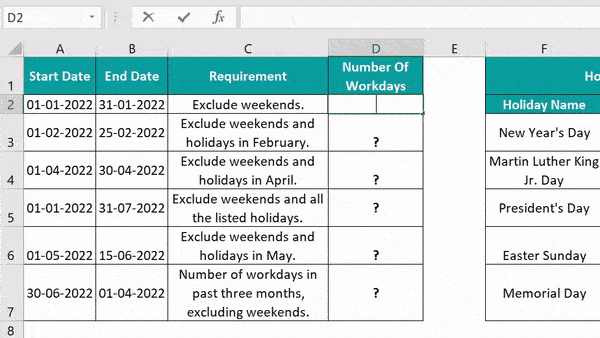[Alternatively, select cell D2, click FormulasDate & TimeNETWORKDAYS.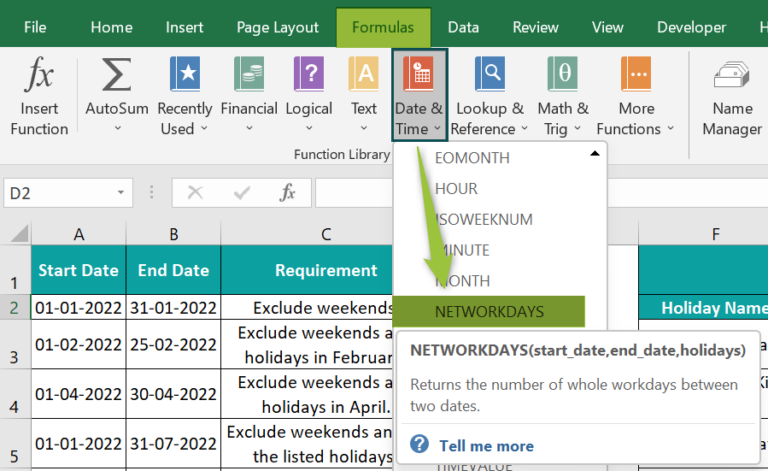The Function Arguments window opens. Enter the argument values as shown below.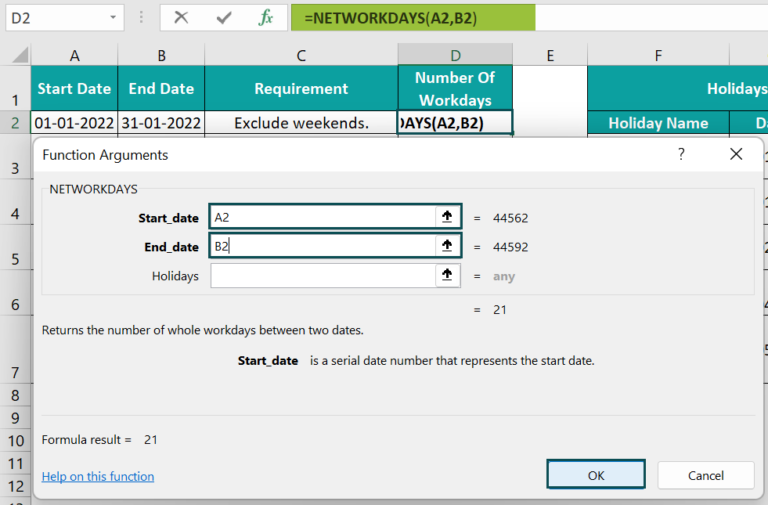Click “OK”.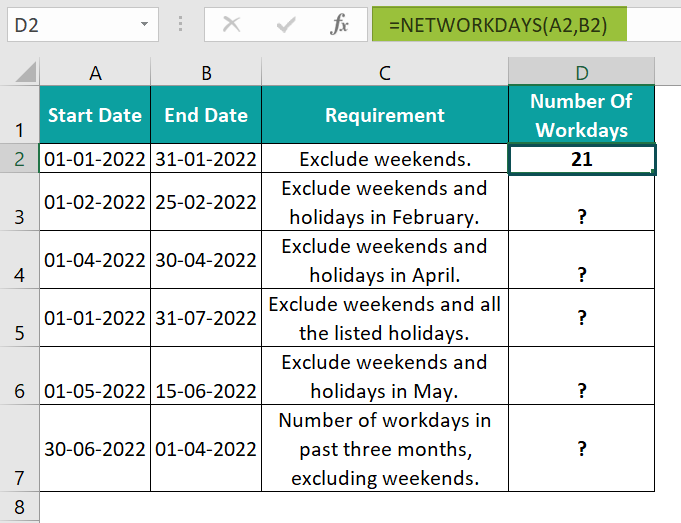2. Select cell D3, enter the formula =NETWORKDAYS(A3,B3,G5), and press the “Enter” key.

[As per the holiday list in the second table, February has one holiday in cell G5. The requirement is to exclude the weekends and holidays in February. So, the function accepts cell references to the given dates in columns A and B, 01-02-2022 and 25-02-2022, and the holiday date, 21-02-2022. And thus, the NETWORKDAYS Excel function return value in the target cell D3 is 18.]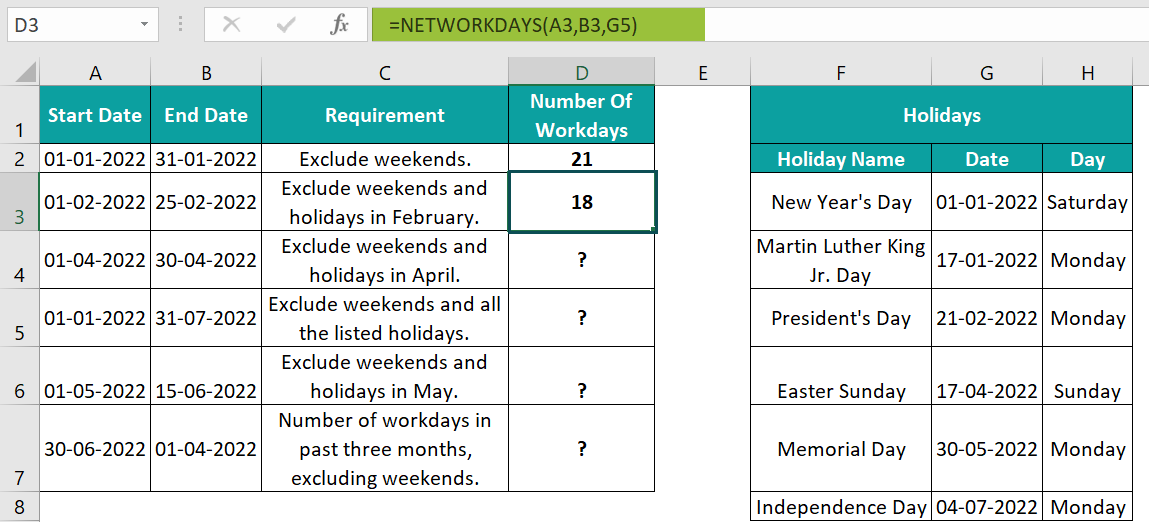3. Next, select cell D4, enter the formula =NETWORKDAYS(A4,B4,G6), and press the “Enter” key.

[Here, the requirement is similar to the previous step, except we need to exclude the holidays in April along with the weekends. But as per the holiday list in the second table, there is one holiday in April. And it falls on a weekend. So, instead of considering the holiday date, 17-04-2022, as a weekend and a holiday, NETWORKDAYS() counts it only as a weekend. And thus, the function excludes nine weekend dates to return the total working days in April as 21.]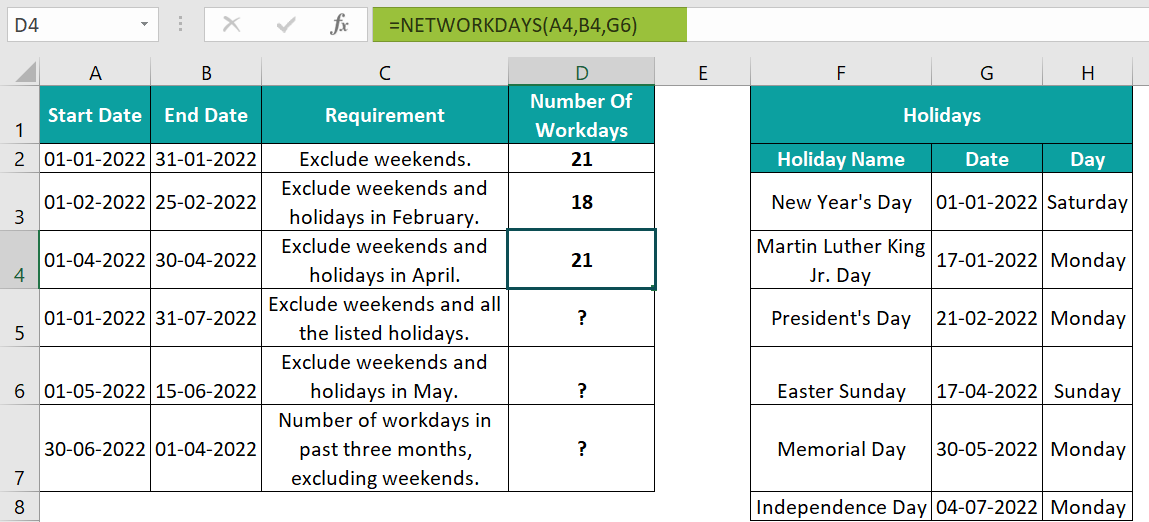4. Select cell D5, enter the formula =NETWORKDAYS(A5,B5,G3:G8), and press the “Enter” key.

[As we need to exclude all the holidays mentioned in the holiday list in the second table, the NETWORKDAYS() takes the argument holidays as a cell range, C3:C8. And as the holidays, 01-01-2022 and 17-04-2022, fall on weekends, the function considers them as weekends. So, it excludes all the weekends between 01-01-2022 and 31-07-2022 and the four holidays listed in the second table, 17-01-2022, 21-02-2022, 30-05-2022, and 04-07-2022. And thus, the function returns the working days count as 146.]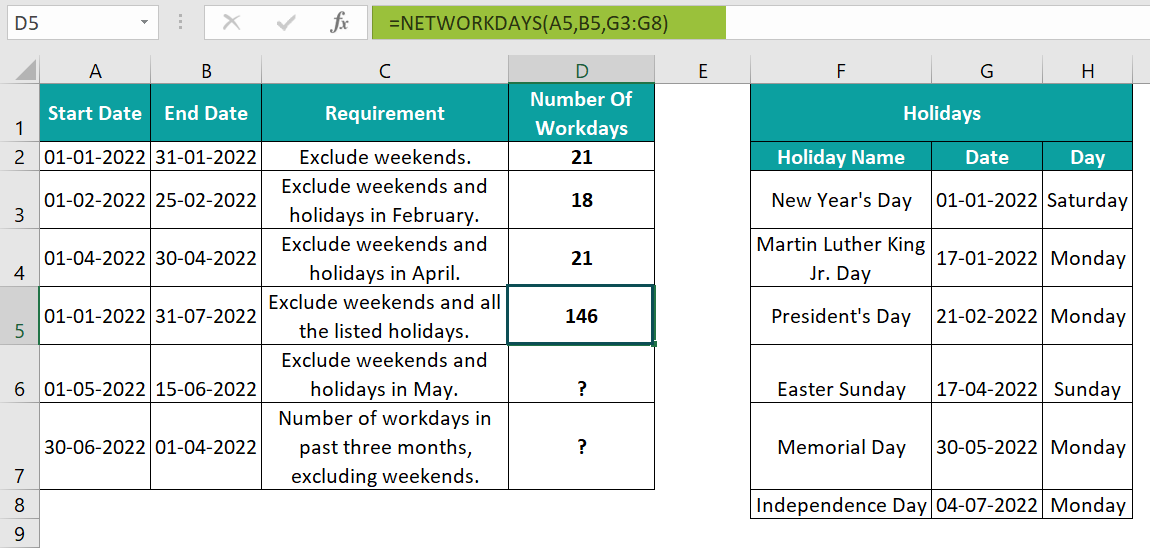5. Then, select cell D6, enter the formula =NETWORKDAYS(“01-05-2022″,”15-06-2022″,”30-05-2022”), and press the “Enter” key.

[Here, the requirement is similar to that explained in step 2. But the above formula shows we can also enter the specific dates in double quotes as the NETWORKDAYS() arguments.]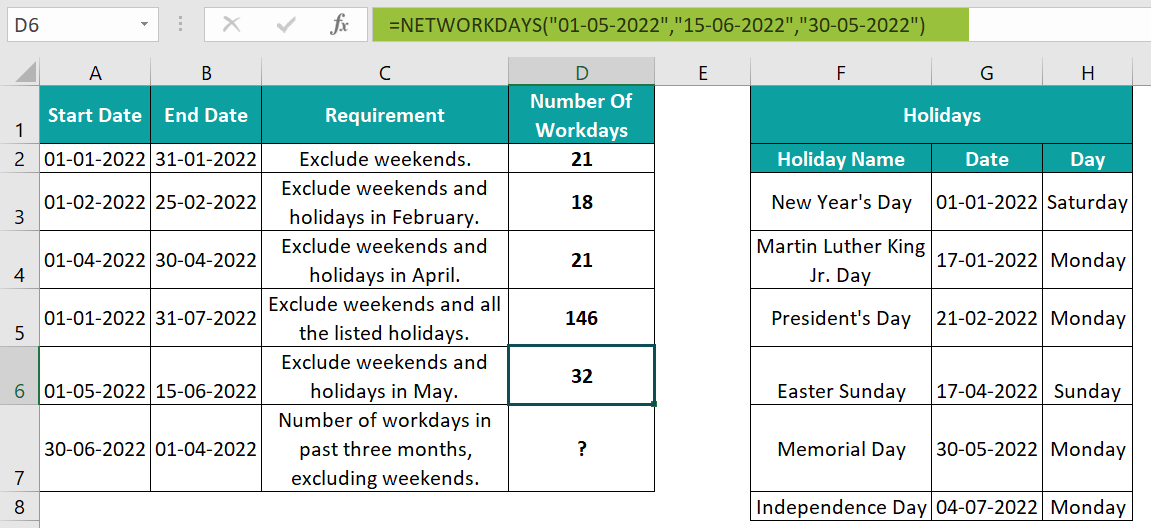6. Select cell D7, enter the formula =NETWORKDAYS(A7,B7), and press the “Enter” key.

[In row 7, the start date falls after the end date. And hence, the NETWORKDAYS Excel function returns a negative number as the count of working days in the specified period, -65.]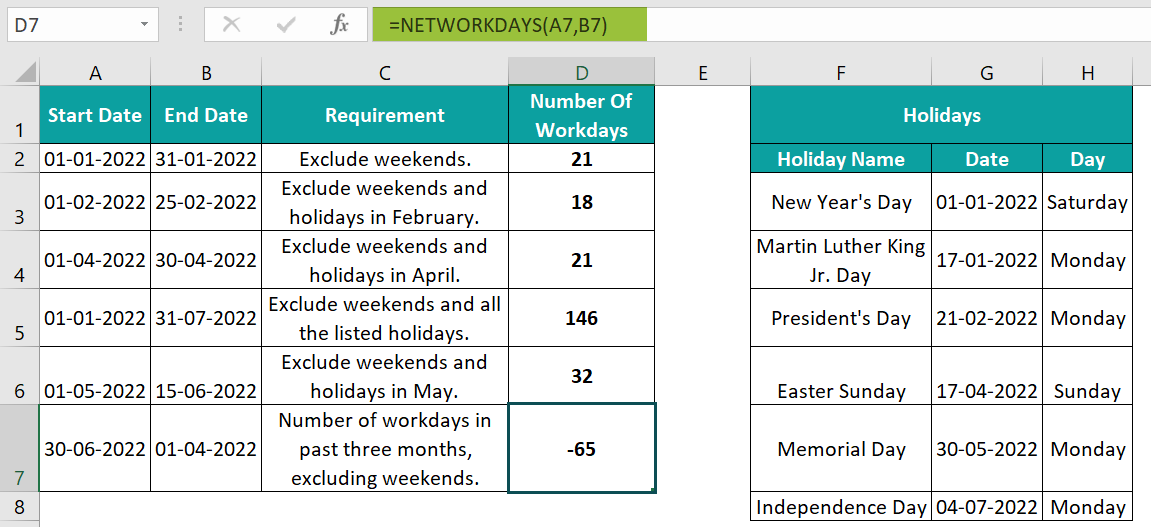Next, we will insert a new row, i.e., row 8, in the first table, as shown below.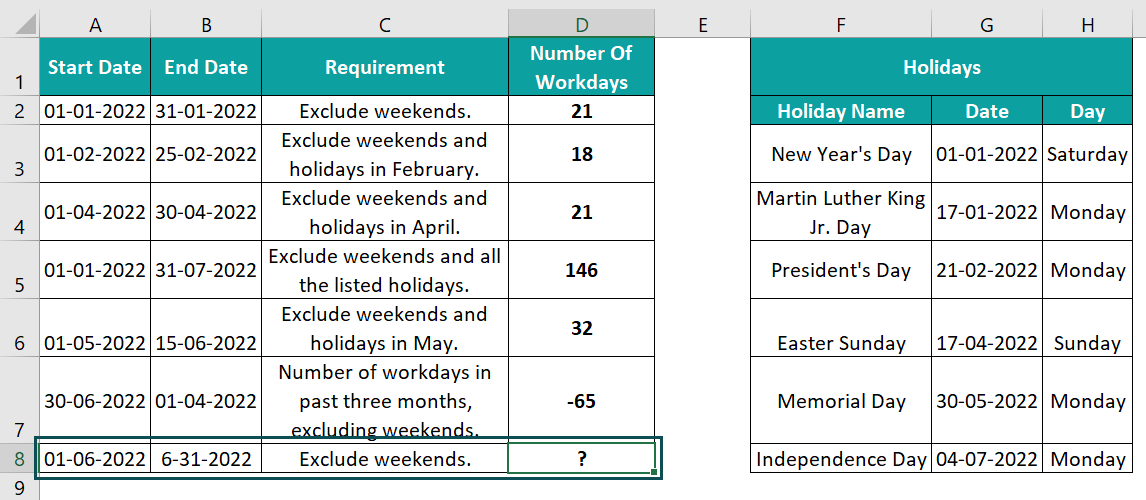7. Finally, select cell D8, enter the formula =NETWORKDAYS(A8,B8), and press the “Enter” key.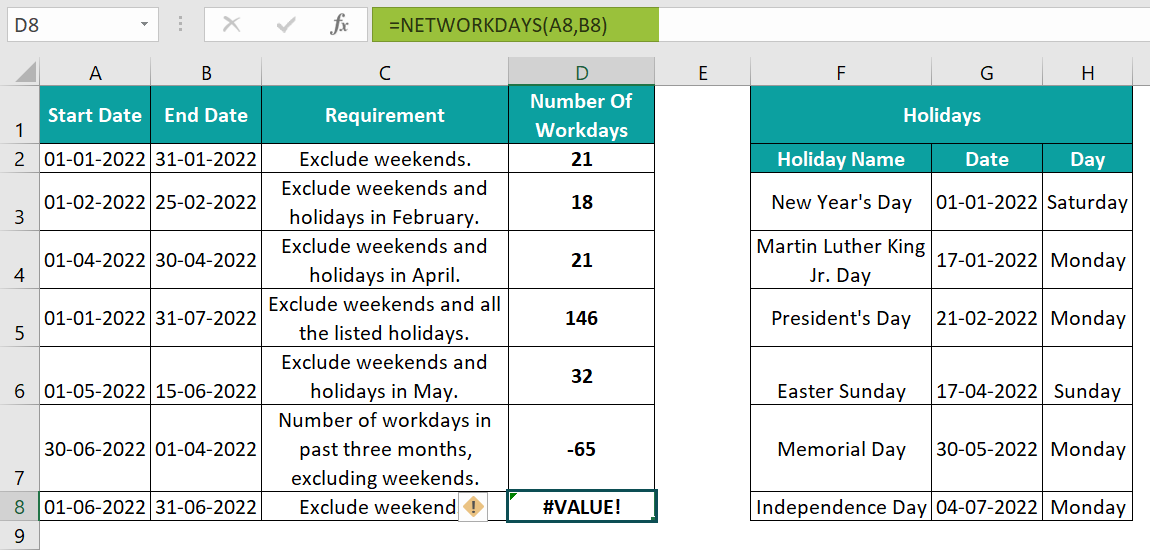The final output is shown above in column D as per the conditions in column C.

[Output Observation: We will get an error when applying the NETWORKDAYS() in cell D8, because cell B8 value, 31-06-2022, provided as the end_date argument, is an invalid date. So, once we correct the cell B8 value, it will eliminate the error.]

### Examples

We will understand some advanced scenarios using NETWORKDAYS Excel function.

#### Example #1

We will calculate the total hours worked during the working days using the NETWORKDAYS Excel function. We must calculate the total number of working days in the given periods and determine the total hours worked on the workdays in each row.

In the below image, the first table shows the first and last working dates and the per-day working hours. And the second table contains the holiday list.

The steps to calculate the total hours using the NETWORKDAYS Excel function are:

• 1: Select cell D2, enter the formula =NETWORKDAYS(A2,B2,\$G\$3:\$G\$5), and press the “Enter” key.
• 2: Next, select cell E2, enter the =D2*C2 formula, and press the “Enter” key.
• 3: Select cells D2:E2 and drag the formulas using the fill handle to the corresponding row 3 cells, D3:E3.

The output is shown above.

[Output Observation: In row 2, as the listed holidays do not fall in the given duration, the NETWORKDAYS() returns the net workdays excluding the weekends, 44. However, in row 3, the three listed holidays fall within the first and last working dates. So, the function excludes them and the weekends to return the net workdays, 40.

And finally, the formulas in cells E2:E3 multiply the calculated net workdays with the per day working hours, 7.5, in each row to achieve the required data.]

#### Example #2

We will calculate the number of workdays between each start date and the month’s end using the NETWORKDAYS Excel function with EOMONTH().

The table below contains a list of start dates for each month from January to December. And we need the monthly net workdays to display them in column B. Assume there is no holiday list, and we need to exclude only weekends.

The steps to calculate the days using the NETWORKDAYS Excel function with EOMONTH() are:

• Step 1: Select cell B2, enter the formula =NETWORKDAYS(A2,EOMONTH(A2,0)), and press the “Enter” key. The result is 21, as shown below.
• Step 2: Drag the formula from cell B2 to B13 using the fill handle.

The output is shown above.

[Output Observation: First, the EOMONTH() returns serial number 44561, representing the last date of December 31-12-2021. And then, the NETWORKDAYS() accepts the start date in cell A13, 1-12-2022, as the first argument and EOMONTH() return value as the second argument. So thus, it returns the total working days in the resulting period excluding weekends, 23.]

#### Example #3

We will calculate the number of workdays between dates using the NETWORKDAYS function along with DATE function, and IF function in excel.

The below image shows three tables. The first table contains the holiday list, and the second shows workday wage conditions. And the third table contains the year for which we must calculate the annual salary based on the given workday calculation and workday wage conditions.

The steps to use NETWORKDAYS(), DATE() and IF() are:

• Step 1: Select cell H6, enter the formula =IF(NETWORKDAYS(DATE(F6,1,1),DATE(F6,12,31))>=251,NETWORKDAYS (DATE(F6,1,1),DATE(F6,12,31))*120,NETWORKDAYS(DATE(F6,1,1),DATE(F6,12,31)) *90), and press the “Enter” key.
• Step 2: Select cell H7, enter the formula =IF(NETWORKDAYS(DATE(F7,1,1),DATE(F7,12,31),B3:B13)>=251,NETWORKDAYS (DATE(F7,1,1),DATE(F7,12,31),B3:B13)*120,NETWORKDAYS(DATE(F7,1,1),DATE (F7,12,31),B3:B13)*90), and press the “Enter” key.

The output is shown above.

[Output Observation: Cell H6 formula accepts only the start and end dates the DATE() returns while ignoring the argument holidays. As a result, it returns the net workdays, 260. And as the IF condition holds, the IF() returns the product of the determined net workdays, 260 and 120, \$31200.

Likewise, the formula in the target cell H7 works similarly. But the only difference is that the NETWORKDAYS() excludes weekends and the nine holidays falling on weekdays in the specified cell range, B3:B13, while calculating the net working days.]

### Important Things to Note

• Ensure the date values we provide to the NETWORKDAYS Excel function have the data format set as Date.
• When the start_date and end_date arguments have the same date value, the NETWORKDAYS() return value is 1, as the function counts the start and end dates.
• We get the #VALUE! error for invalid argument values.

1. Where is the NETWORKDAYS function in Excel?

The NETWORKDAYS function in Excel is in the Formulas tab. Click Formulas Date & TimeNETWORKDAYS.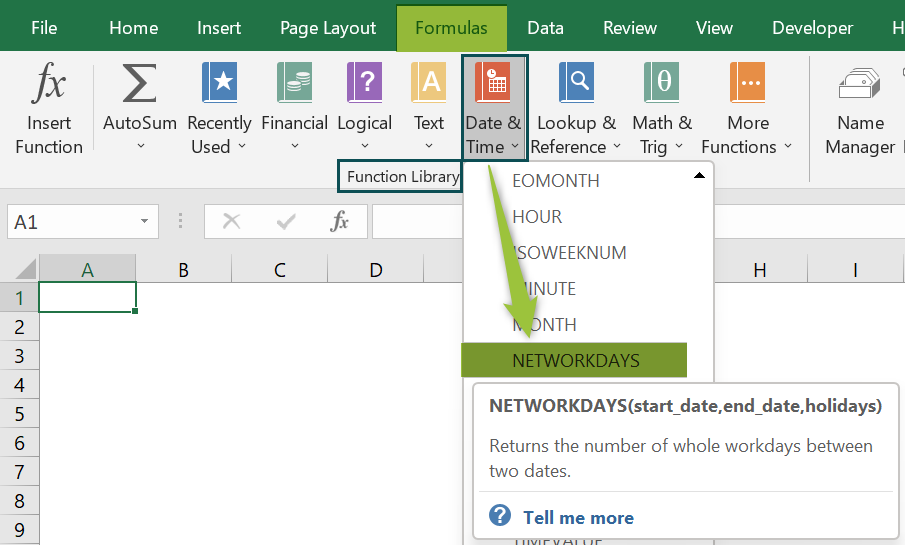2. Does the NETWORKDAYS() include weekends and holidays?

The NETWORKDAYS() does not include weekends by default. The functions excluded the holidays when specified while calculating the workdays between two given dates.

3. How to use NETWORKDAYS in Excel VBA?

We can use NETWORKDAYS in Excel VBA using the method:

Application.WorksheetFunction.NETWORKDAYS(start_date,end_date,holidays)
Let us see how to calculate the net workdays in the duration specified in each row using NETWORKDAYS and VBA with an example.

The following table contains the start and end dates in columns A and B and holidays in column C.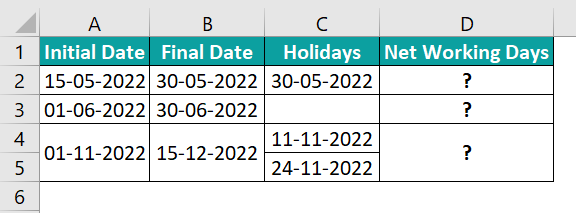The steps to using the NETWORKDAYS in VBA are:

• 1: In the current worksheet, press the shortcut keys Alt + F11 to open the VBA Editor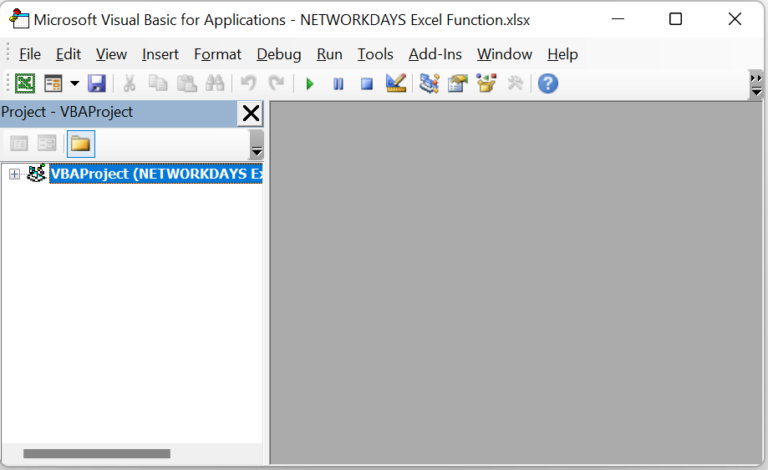• 2: Then choose the required VBAProject and select InsertModule in the top menu to open the Module1 window.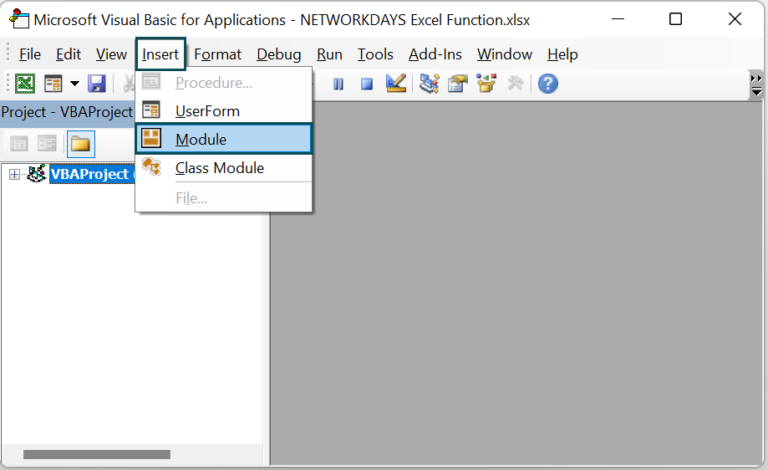• 3: Enter the VBA code, shown in the below image, in the module 1 window to apply the NETWORKDAYS() on the specific target cells.

Sub NETWORKDAYS_fn()
Range(“D2”) = Application.WorksheetFunction.NetworkDays(Range(“A2”), range(“B2”), Range(“C2”))
Range(“D3”) = Application.WorksheetFunction.NetworkDays(Range(“A3”), range(“B3”))
Range(“D4”) = Application.WorksheetFunction.NetworkDays(Range(“A4”), range(“B4”), Range(“C4:C5”))
End Sub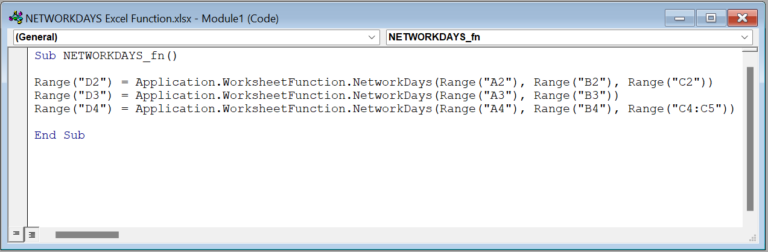• 4: Click the Run Sub/UserForm icon to run the Module1 code.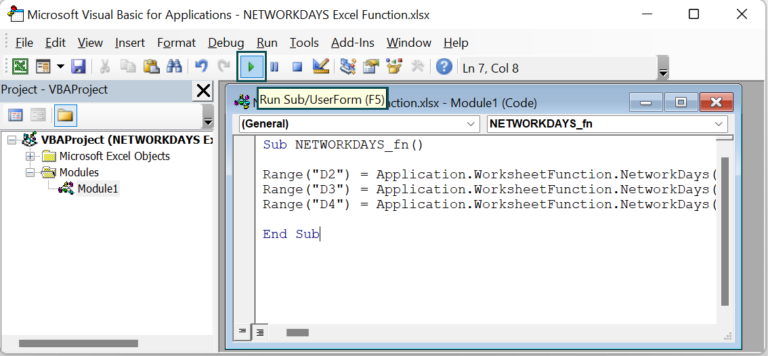Finally, if we open the active worksheet, we will see the NETWORKDAYS() executed and the required results in the target cells D2:D4.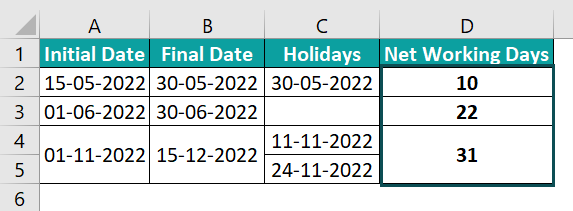[Please Note: In row 2, the final date and holiday are the same. However, as the holiday is a weekday, the NETWORKDAYS() counts it as a holiday and excludes it along with the weekends while calculating the net workdays.]